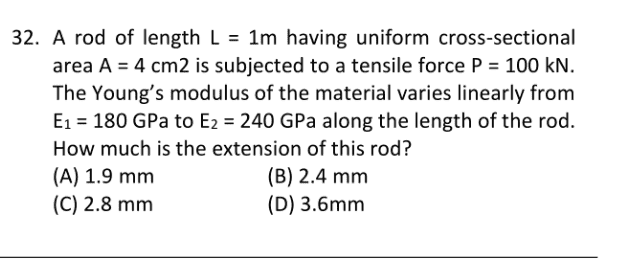# Extension of rod -- help please

## Homework Statement

see attachementdl= PL/AE

## The Attempt at a Solution

as you see here my youngs modulus E varies linearly. i doubt that adding up E1 &E2 wld give solution.. any help?

Last edited by a moderator:

mfb
Mentor
What is the (approximate) Young's modulus in the first centimeter? How much will it get stretched?
What is the (approximate) Young's modulus in the last centimeter? How much will it get stretched?
Instead of calculating it centimeter by centimeter, how can you get an "exact" solution?

Chestermiller
Mentor
What is the tensile stress in the rod? Do you know the relationship between strain and displacement u?

Instead of calculating it centimeter by centimeter, how can you get an "exact" solution?
centimeter by centimeter?. well threre must be some other way, like its going to be triangular profile with one end E1 and other end E2.. is that possible to find equivalent E acting on rod ..

What is the tensile stress in the rod? Do you know the relationship between strain and displacement u?
yes sir .tensile stress =P/A. and strain = dL/L. .by hookes law E=stress/strain giives dL= PL/AE

mfb
Mentor
centimeter by centimeter?. well threre must be some other way, like its going to be triangular profile with one end E1 and other end E2.. is that possible to find equivalent E acting on rod ..
The centimeter by centimeter calculation is not correct, but if you answer my questions it should give you an idea how the correct approach looks like.
It is not the first question I am helping at, I suggest that for a good reason.

Chestermiller
Mentor
yes sir .tensile stress =P/A. and strain = dL/L. .by hookes law E=stress/strain giives dL= PL/AE
Your equation for the strain is valid only if it is uniform from one end of the rod to the other. In this problem, because E varies with position along the rod, the strain is not uniform from one end to the other. So you need to figure out how to handle a problem in which the strain is varying with position along the rod.

Let x represent axial position measured along the rod before the rod is placed under load. Let the end x = 0 be fixed, and let the end x = L be where the load P is applied. We are going to focus on one cross section of the rod at location x before the rod is placed under load. After the rod is placed under load, this same cross section has moved to a new axial location at x + u(x), where u(x) is the distance that the cross section originally at x has moved. We call u(x) the displacement of the cross section. Of course, since the end at x = 0 is fixed, u(0) = 0.

Now let's look at what happens to two closely neighboring adjacent cross sections of the rod that were initially located at x and at x + Δx. What was the original distance between these two cross sections? What is the distance between these same two cross sections after the load has been applied? What is the increase in distance between the two cross sections? What is the increase in distance divided by the original distance? What is the limit of this as Δx approaches zero?

Chet

well then the material vary at every point (as u said).. so i need to find extension at given small dx. and integrate from 0 to L . is that right

Chestermiller
Mentor
well then the material vary at every point (as u said).. so i need to find extension at given small dx. and integrate from 0 to L . is that right

Chet

only problem im facing is its E value .. at dx E= E1-((E2-E2)/L)*x .im bit confused.

Chestermiller
Mentor
only problem im facing is its E value .. at dx E= E1-((E2-E2)/L)*x .im bit confused.

Chet

let original lenght be L ... but i have no idea how to find lenght btw consecutive section after load is applied.

It is not the first question I am helping at, I suggest that for a good reason.
i didnot mean that way. i value you all. but i was in a hurry,, needed aproach or formula that i miss.exams coming up.

Mark44
Mentor
i didnot mean that way. i value you all. but i was in a hurry,, needed aproach or formula that i miss.exams coming up.
Several people have laid out an approach, but you need to come up with a way to use what they have said. We are not going to give you a formula that you can blindly plug numbers into.

Chestermiller
Mentor
let original lenght be L ... but i have no idea how to find lenght btw consecutive section after load is applied.

Distance between successive cross sections after load is applied =## [x + Δx + u(x+Δx)]-[x + u(x)] = Δx + u(x+Δx) - u(x)##

This is my last hint until I see some effort on your part.

Chet

yea
Several people have laid out an approach, but you need to come up with a way to use what they have said. We are not going to give you a formula that you can blindly plug numbers into.
h .yeah ,.. im seeing it ,anyway thanks

Chestermiller
Mentor
yea

h .yeah ,.. im seeing it ,anyway thanks
Not so fast. So, please show us your solution. It's the least you can do, given that we spent so much of our (valuable) time trying to help you.

Chet